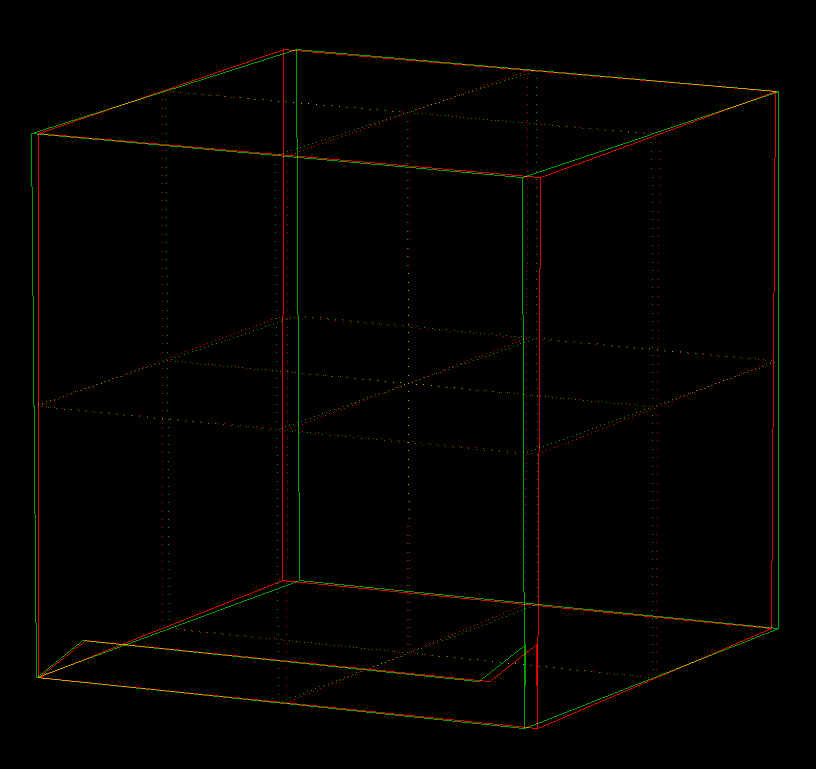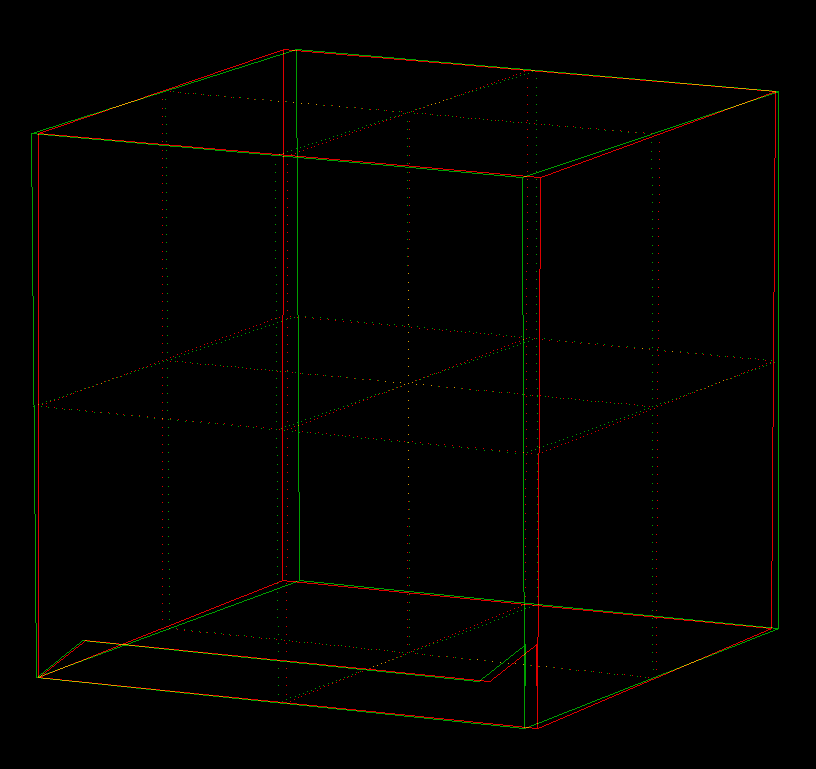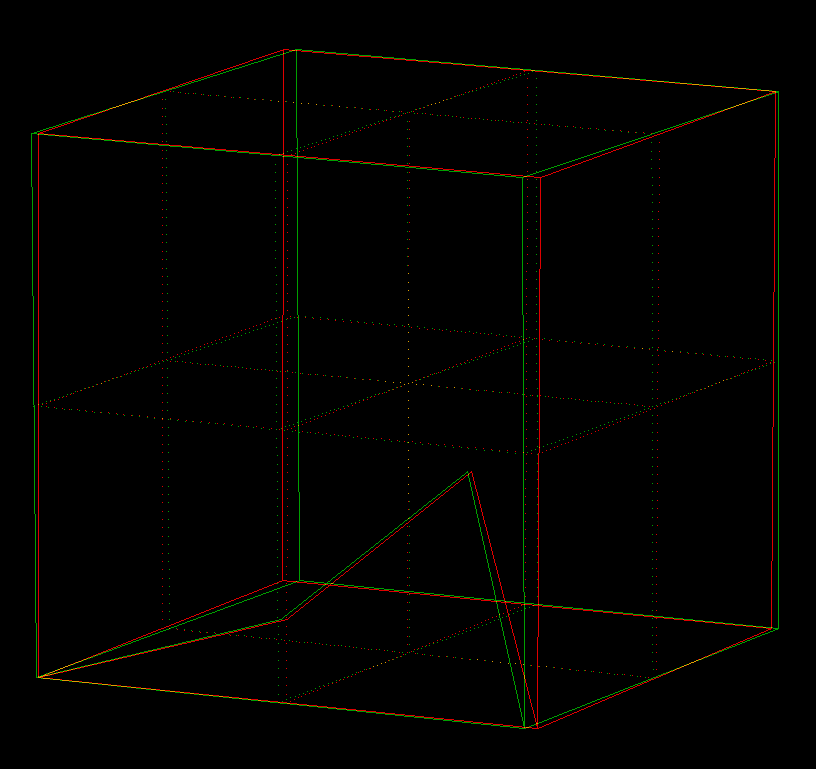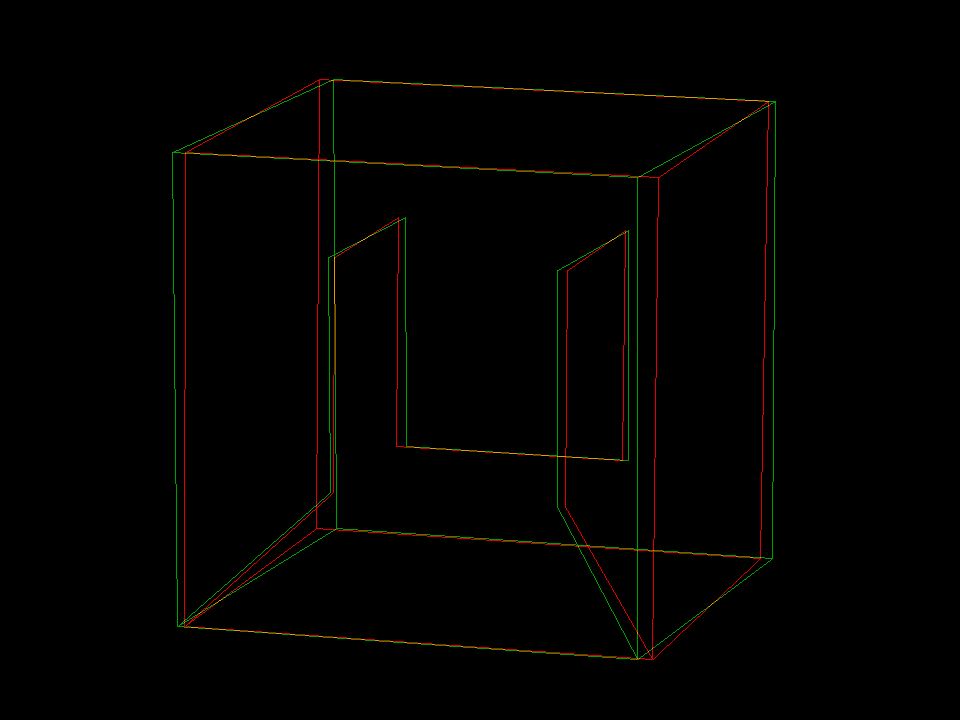# Hilbert's Cube-Filling Curvehilbert cubefill Hilbert's square filling continuous curve can easily be generalized to 3 (and more) dimensions. Begin with some curve, inside a cube, from the front-left-bottom corner to the front-right-bottom corner. Next scale the cube with the initial curve down by a factor 1/2 and make 8 copies of this. The 8 small cubes of course fit into the initial cube - but it has to be done in such a way that the 8 small curves fit together to a new curve from front-left-bottom to front-right-bottom, see next image. Now repeat.hilbert cube construct These two images show the initial curve and the first iteration in the subdivided cube. The initial curve has a spike near its end, so that one can see that the 8 scaled down curves fit together without changing the direction in which they are traversed. The four top copies are only rotated, the four bottom copies are reflections of the scaled down copies. The maximal distance which any point in the cube has from the image of the curve decreases by a factor 2 (at least) in each iteration. The limit curve, therefore, passes through all points of the cube.hilbert cube 5construc Five iterations of another (non-symmetric) initial curve. Note that the limit curve is independent of the initial curve; it depends only on the iteration procedure and the iteration of the two endpoints of the initial curve.hilbert cube 5iterate 001hilbert cube filling curve

Hilbert_CubeFillCurve.pdf